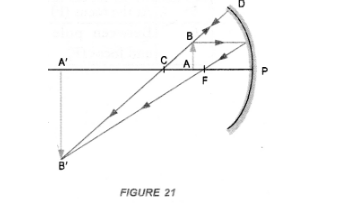# Under which of the following conditions

Question:

Under which of the following conditions a concave mirror can form a real image larger than the actual object ?

(a) When object is kept at a distance equal to its radius of curvature.

(b) When object is placed between the focus and centre of curvature.

(c) When object is kept at a distance less than its focal length.

(d) When object is kept at a distance greater than its radius of curvature.

Solution:

(b).
Explanation :